Jai Sachith Paul worked at EFY till recently. The article is based on a talk given by P. Chow Reddy, managing director, Interleaved Technologies, at Electronics Rocks Conference 2014

When it comes to the embedded world, the microcontroller can perform a very large number of distinct functions. The performance of the controller for complex mathematical computations is limited by its speed. Digital signal processors (DSPs) are always an option for improved performance, but their use increases system complexity and cost of the project. Here comes the relevance of Vedic Mathematics. Logical calculations based on sutras in Vedic Mathematics can simplify computations, and significantly improve processing speed and performance of the controller.

Incorporating Vedic Mathematics into microcontrollers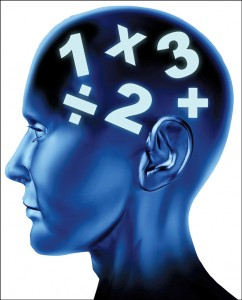In systems such as industrial process controllers, there are a good number of calculations to be done. Here, the process should be controlled based on the output of these calculations. Therefore the time required by the microcontroller for these calculations is very critical.

We can consider a microcontroller as a clone of a human being in terms of calculations. Like a human being, a microcontroller can also perform calculations logically and in lesser time. Logics needed for these calculations are readily available from the sutras, which can be programmed into the application and made as a routine that can be called any time for calculating values. Based on logical decision, the controller selects which routine is to be called.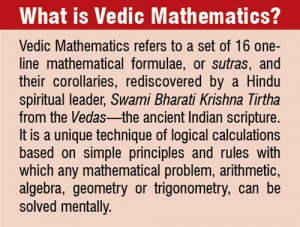By using this technique, an increase in speed up to ten or even 15 times the original can be achieved. “A code written on 8051 series of microcontroller (using Vedic Mathematics) with 12MHz crystal to perform square of a two-digit number takes only 18 microseconds to generate an answer. Any other faster microcontroller, or even a PC, takes more than 200 microseconds in general mathematical methods. This almost eliminates the need of DSP microcontrollers,” says P. Chow Reddy of Interleaved Technologies, Hyderabad.

Applying Vedic Mathematics
The idea can be adapted to various applications for making these faster and smoother. Let us take a look at some interesting applications.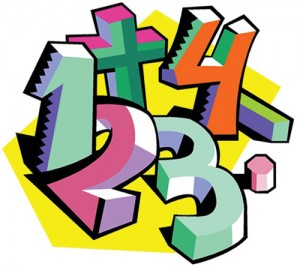Mobile applications. Applications in Android should be efficient so as not to interfere in the telephony. But this is not the case today. If we try to find the factorial of a number (say, 69) using the scientific calculator in an Android device, it will take almost one second. The performance in this case is seriously affected. We can make use of logical calculations from Vedic Mathematics so as to modify the code and improve the speed of computation.

Transformer winding machines. Conventionally, we have transformer winding machines that take data input of parameters, such as primary number of turns and gauge, secondary number of turns and gauge, bobbin and the code. An expert should be made available to gather and feed data. Even in this case, we can program an 8051 microcontroller to automate transformer winding. If we make use of routines based on sutras, we can increase the speed of this microcontroller, which is a critical factor in transformer winding.

Aerofoil controllers. Aerofoil has a finite number of sensors that gather and send data to the laptop. Here, computations are made and the inclination needed is sent back to activators. The idea is to replace the laptop with a microcontroller that makes use of Vedic Mathematics to improve the speed of computations.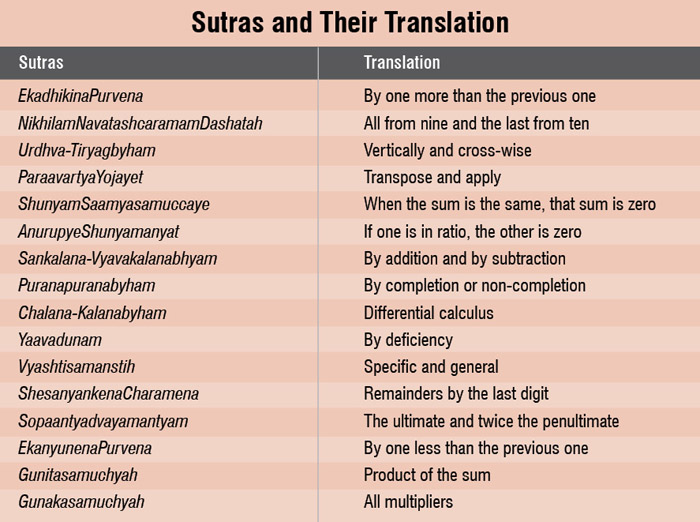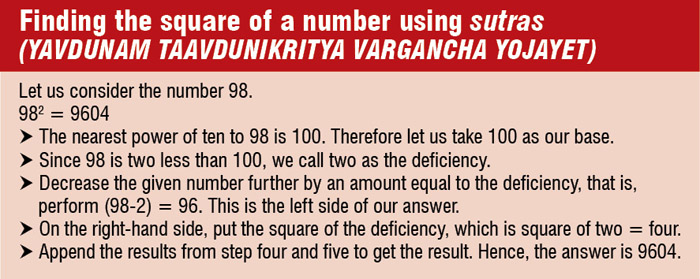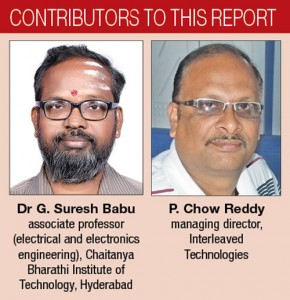Deciphering the algorithm. The main difficulty in materialising this concept is in gathering information from the Vedas, which is written in Sanskrit. The challenge is to read and decipher the algorithm encrypted in the scripture. If this is achieved, the engineer can easily code it into microcontrollers.

Efficient coding. In order to make the code short and efficient, it should be written in an assembly language—a language that both a microcontroller and programmer understand. Opting for C language for easier coding will consume a lot of program memory. Thus, writing an efficient code is necessarily troublesome for the engineer, but beneficial for users of the product.

What the future holds
The idea of incorporating Vedic knowledge of Mathematics into microcontrollers was developed by P. Chow Reddy in collaboration with Dr G. Suresh Babu, associate professor in electrical and electronics engineering, Chaitanya Bharathi Institute of Technology, Hyderabad. This concept has the potential of revolutionising the embedded industry by improving the performance of microcontrollers by 10-15 folds. Deciphering the algorithm from Vedas still remains a major challenge.

1.Vasantha
2.Sanevasantha
•PEDDI CHOW REDDY